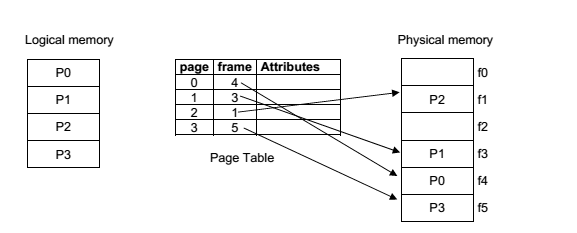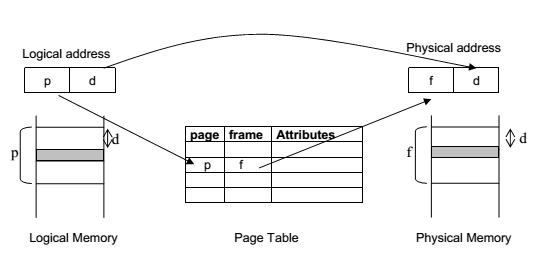Why Paging is Required ?

Paging permits a program to allocate noncontiguous blocks of memory. The OS divide programs into pages which are blocks of small and fixed size. Then, it divides the physical memory into frames which are blocks of size equal to page size. The OS uses a page table to map program pages to memory frames. Page size (S) is defined by the hardware. Generally page size is chosen as a power of 2 such as 512 words/page or 4096 words/page
etc.

With this arrangement, the words in the program have an address called as logical address.

Every logical address is formed of

•  A page number p where p = logical address div S
•  An offset d where d = logical address mod S

When a logical address <p, d> is generated by the processor, first the frame number f corresponding to page p is determined by using the page table and then the physical address is calculated as (f*S+d) and the memory is accessed.The address translation in paging is shown below